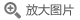## 量子系统的非平衡多体理论(英文版)

• 定价： ￥129
• ISBN：9787519264192
• 开 本：16开 平装
• 作者：(意)G.斯蒂芬尼茨...
• 立即节省：
• 2019-09-01 第1版
• 2019-09-01 第1次印刷### 导语### 内容提要格林函数是解决物理学问题最强大和最通用的方法之一，其中的非平衡态理论对许多研究领域的影响更是不可估量的。本书自成体系，全面论述了非平衡态多体理论。作者从量子力学入手，阐述了平衡态和非平衡态格林函数形式，轮廓格林函数和图解展开式的物理内涵，介绍了这些理论在从分子、纳米结构到金属和绝缘体等诸多领域的应用。本书适用于物理及相关专业的研究生和科研工作者。

### 目录

Preface
List of abbreviations and acronyms
Fundamental constants and basic relations
1  Second quantization
1.1   Quantum mechanics of one particle
1.2  Quantum mechanics of many particles
1.3  Quantum mechanics of many identical particles
1.4   Field operators
1.5   General basis states
1.6   Hamiltonian in second quantization
1.7   Density matrices and quantum averages
2  Getting familiar with second quantization: model Hamiltonians
2.1   Model Hamiltonians
2.2  Pariser-Parr-Pople model
2.3  Noninteracting models
2.3.1   Bloch theorem and band structure
2.3.2   Fano model
2.4  Hubbard model
2.4.1   Particle-hole symmetry: application to the Hubbard dimer
2.5  Heisenberg model
2.6  BCS model and the exact Richardson solution
2.7  Holstein model
2.7.1   Peierls instability
2.7.2   Lang-Firsov transformation: the heavy polaron
3  Time-dependent problems and equations of motion
3.1   Introduction
3.2  Evolution operator
3.3  Equations of motion for operators in the Heisenberg picture
3.4  Continuity equation: paramagnetic and diamagnetic currents
3.5  Lorentz Force
4 The contour idea
4.1   Time-dependent quantum averages
4.2  Time-dependent ensemble averages
4.3  Initial equilibrium and adiabatic switching
4.4  Equations of motion on the contour
4.5  Operator correlators on the contour
5    Many-particle Green's functions
5.1   Martin-Schwinger hierarchy
5.2  Truncation of the hierarchy
5.3  Exact solution of the hierarchy from Wick's theorem
5.4  Finite and zero-temperature formalism from the exact solution
5.5  Langreth rules
6  One-particle Green's function
6.1   What can we learn from G?
6.1.1    The inevitable emergence of memory
6.1.2   Matsubara Green's function and initial preparations
6.1.3   Lesser/greater Green's function: relaxation and quasi-particles
6.2  Noninteracting Green's function
6.2.1   Matsubara component
6.2.2   Lesser and greater components
6.2.3   All other components and a useful exercise
6.3  Interacting Green's function and Lehmann representation
6.3.1   Steady-states, persistent oscillations, initial-state dependence
6.3.2   Fluctuation-dissipation theorem and other exact properties
6.3.3   Spectral function and probability interpretation
6.3.4   Photoemission experiments and interaction effects
6.4  Total energy from the Galitskii-Migdal formula
7  Mean field approximations
7.1   Introduction
7.2  Hartree approximation
7.2.1   Hartree equations
7.2.2   Electron gas
7.2.3   Quantum discharge of a capacitor
7.3  Hartree-Fock approximation
7.3.1   Hartree-Fock equations
7.3.2   Coulombic electron gas and spin-polarized solutions
8  Conserving approximations: two-particle Green's function
8.1   Introduction
8.2  Conditions on the approximate G2
8.3  Continuity equation
8.4  Momentumconservation law
8.5  Angular momentum conservation law
8.6  Energy conservation law
9  Conserving approximations: self-energy
9.1   Self-energy and Dyson equations I
9.2  Conditions on the approximate Σ
9.3  φ functional
9.4  Kadanoff-Baym equations
9.5  Fluctuation-dissipation theorem for the self-energy
9.6  Recovering equilibrium from the Kadanoff-Baym equations
9.7  Formal solution of the Kadanoff-Baym equations
10 MBPT for the Green's function
10.1  Getting started with Feynman diagrams
10.2  Loop rule
10.3  Cancellation of disconnected diagrams
10.4  Summing only the topologically inequivalent diagrams
10.5  Self-energy and Dyson equations II
10.6  G-skeleton diagrams
10.7  W-skeleton diagrams
10.8  Summary and Feynman rules
11 MBPT and variational principles for the grand potential
1l.l   Linked cluster theorem
11.2  Summing only the topologically inequivalent diagrams
11.3  How to construct the φ functional
11.4  Dressed expansion of the grand potential
11.5  Luttinger-Ward and Klein functionals
11.6  Luttinger-Ward theorem
11.7  Relation between the reducible polarizability and the ~ functional
11.8  Ψ functional
11.9  Screened functionals
12 MBPT for the two-particle Green's function
12.1  Diagrams for G2 and loop rule
12.2  Bethe-Salpeter equation
12.3  Excitons
12.4  Diagrammatic proof of K = ±δΣ/δG
12.5  Vertex function and Hedin equations
13 Applications of MBPT to equilibrium problems
13.1  Lifetimes and quasi-particles
13.2  Fluctuation-dissipation theorem for P and W
13.3  Correlations in the second-Born approximation
13.3.1   Polarization effects
13.4  Ground-state energy and correlation energy
13.5  GW correlation energy of a Coulombic electron gas
13.6  T-matrix approximation
13.6.1   Formation of a Cooper pair
14 Linear response theory: preliminaries
14.1  Introduction
14.2  Shortcomings of the linear response theory
14.2.1   Discrete-discrete coupling
14.2.2  Discrete-continuum coupling
14.2.3  Continuum-continuum coupling
14.3  Fermi golden rule
14.4  Kubo formula
15 Linear response theory: many-body formulation
15.1  Current and density response function
15.2  Lehmann representation
15.2.1   Analytic structure
15.2.2  The f-sum rule
15.2.3  Noninteracting fermions
15.3  Bethe-Salpeter equation from the variation of a conserving G
15.4  Ward identity and the f-sum rule
15.5  Time-dependent screening in an electron gas
15.5.1   Noninteracting density response function
15.5.2  RPA density response function
15.5.3  Sudden creation of a localized hole
15.5.4  Spectral properties in the GoWo approximation
16 Applications of MBPT to nonequilibrium problems
16.1  Kadanoff-Baym equations for open systems
16.2  Time-dependent quantum transport: an exact solution
16.2.1   Landauer-Büttiker formula
16.3  Implementation of the Kadanoff-Baym equations
16.3.1   Time-stepping technique
16.3.2  Second-Born and GW self-energies
16.4  Initial-state and history dependence
16.5  Charge conservation
16.6  Time-dependent GW approximation in open systems
16.6.1   Keldysh Green's functions in the double-time plane
16.6.2  Time-dependent current and spectral function
16.6.3  Screened interaction and physical interpretation
16.7  Inbedding technique: how to explore the reservoirs
16.8  Response functions from time-propagation
Appendices
A From the N roots of ! to the Dirac δ-function
B Graphical approach to permanents and determinants
C Density matrices and probability interpretatio
D Thermodynamics and statistical mechanics
E Green's functions and lattice symmetry
F Asymptotic expansions
G Wick's theorem for general initial states
H BBGKY hierarchy
I From δ-like peaks to continuous spectral functions
J Virial theorem for conserving approximations
K Momentum distribution and sharpness of the Fermi surface
L Hedin equations from a generating functional
M Lippmann-Schwinger equation and cross-section
N Why the name Random Phase Approximation~
O Kramers-Kronig relations
P Algorithm for solving the Kadanoff-Baym equations
References
Index